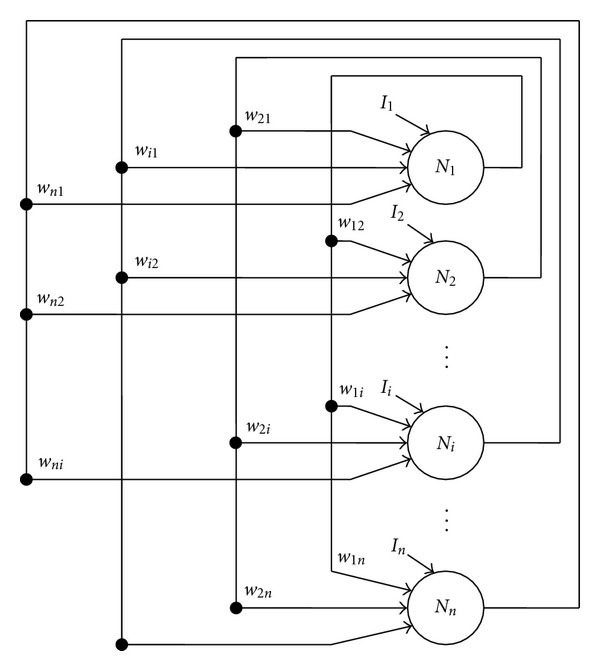Search
• Dr. Hatem Hassanin

# Solving Optimization Problems Using Hopfield Neural Network

The optimization is the process of selecting the best option of all. A large number of tasks reduced to optimization problems: the unconstrained optimization of nonlinear functions; method of least squares; solution of nonlinear equations; linear programming; quadratic programming; conditional minimization of nonlinear functions; mini max methods; multi-objective optimization.Discrete Hopfield neural network.

In the light of these provisions, the relevance of this work is evident for the need to address solving optimization problems using neural network. The implementation of numerical algorithms implemented by software Matlab. A neural network is a system of "neurons", interacting with each other like this neural network of the brain. "Neuron" in this case, it is a kind of having state of the computational process, so that the network can operate in parallel. Artificial network "learns" the solution of certain problems that, in fact, reduced to the computation of the weight coefficients of the matrix, without any "magic".

With the help of neural networks, we try to solve various problems. The purpose of writing this paper is to study the solution of optimization problems using the Hopfield neural network.

Keywords: Optimization, hemispherical, stable point, the basin of attraction, Hopfield neural network.

1 view0 comments

See All